Vectors

Introduction

This topic introduces you to basic concepts in analytic geometry. Recall from FP1 and FP2 that a vector is a matrix with dimension $1\times n$ or $n\times 1$, i.e. it either has one column or one row. Conceptually a vector can be thought of as a straight line in space pointing in a direction.

In FP3 we are concerned with vectors and planes in $\mathbb{R}^3$. The study of vectors in three-dimensional space has a wide variety of applications in physics and engineering, and forms a basis for the study of linear algebra in undergraduate mathematics. I'm going to start this section by going through some definitions and revision points.

A set $V$ of elements, which we call vectors, is a vector space if in $V$ there are defined two algebraic operations called vector addition and scalar multiplication.

For vector addition the following axioms must hold

• Commutativity: $$\mathbf{a}+\mathbf{b} = \mathbf{b}+\mathbf{a}$$
• Associativity: $$\left(\mathbf{a}+\mathbf{b}\right)+\mathbf{c} = \mathbf{a}+\left(\mathbf{b}+\mathbf{c}\right)$$
• Existence of a unique zero vector: $$\mathbf{a}+\mathbf{0} = \mathbf{a}$$
• Existence of an additive inverse $-\mathbf{a}$ for each vector $\mathbf{a}$: $$\mathbf{a}+\left(-\mathbf{a}\right) = \mathbf{0}$$

For scalar multiplication the following axioms must hold

• Distributivity: $$c\left(\mathbf{a}+\mathbf{b}\right) = c\mathbf{a}+c\mathbf{b}$$ $$\left(c+d\right)\mathbf{a} = c\mathbf{a}+d\mathbf{a}$$
• Associativity: $$c\left(d\mathbf{a}\right) = \left(cd\right)\mathbf{a}$$
• Identity: $$1\mathbf{a} = \mathbf{a}$$

Recall that multiple vectors are linearly independent if they are not scalar multiples of each other, or if they can't be formed by adding together scalar multiples of each other. They are linearly dependent if the reverse is true.

The dot product of two vectors is a scalar quantity calculated as $$\mathbf{a}\cdot\mathbf{b} = \mathbf{a}^{\textrm{T}}\mathbf{b} = \left[\begin{array}{ccc} a_1 & \ldots & a_n \end{array}\right]\left[\begin{array}{c} b_1 \\ \vdots \\ b_n \end{array} \right] = a_1 b_1 + \ldots + a_n b_n$$

The dot product is linear, meaning $$\left(c\mathbf{a}+d\mathbf{b}\right)\cdot\mathbf{p} = c\left(\mathbf{a}\cdot\mathbf{p}\right)+d\left(\mathbf{b}\cdot\mathbf{p}\right)$$ and it is symmetric $$\mathbf{a}\cdot\mathbf{b} = \mathbf{b}\cdot\mathbf{a}$$ and finally the positive-definite property $$\mathbf{a}\cdot\mathbf{a} \ge 0$$ with equality if and only if the vector is $\mathbf{0}$ $$\mathbf{a}\cdot\mathbf{a} = 0 \iff \mathbf{a} = \mathbf{0}$$

Informally I like to think of the dot product of two vectors as their "in-common-ness". If two vectors have very similar direction then their dot product is larger. You will see in a second why this concept makes sense. Indeed the dot product of two orthogonal vectors (at 90 degrees to each other) is always zero $$\mathbf{a}\cdot \mathbf{b} = 0$$

The norm of a single vector is its length, which can be calculated using the dot product with itself $$\|\mathbf{a}\| = \sqrt{\mathbf{a}\cdot\mathbf{a}} = \sqrt{a_1^2 + \ldots + a_n^2}$$ which is always $\ge 0$, helping the positive-definite property above make more sense. For some vector $\mathbf{a}$ the unit vector in the same direction is $$\hat{\mathbf{a}} = \frac{\mathbf{a}}{\|\mathbf{a}\|}$$

Another way to calculate the dot product of two vectors uses the norms of both the vectors $$\mathbf{a}\cdot\mathbf{b} = \|\mathbf{a}\|\|\mathbf{b}\|\cos \theta$$ where $\theta$ is the angle between them. Note that by rearranging the above formula we can calculate this angle between the two vectors $$\cos \theta = \frac{\mathbf{a}\cdot\mathbf{b}}{\|\mathbf{a}\|\|\mathbf{b}\|} \Rightarrow \theta = \cos^{-1}\frac{\mathbf{a}\cdot\mathbf{b}}{\|\mathbf{a}\|\|\mathbf{b}\|}$$

While we're on the subject of vector norms, note the triangle inequality $$\|\mathbf{a}+\mathbf{b}\| \le \|\mathbf{a}\| + \|\mathbf{b}\|$$

To prove this, think of the following question. If you want to visit your grandmother, which way is the shortest?

1. Walk there in a straight line
2. Walk to the shop first and then to your grandmother's house

The vector cross product

The cross product of two linearly independent vectors is a vector perpendicular to both of them.

The cross product of two vectors is the determinant of a $3\times 3$ matrix formed by the two vectors and the standard basis vectors $\mathbf{i}$, $\mathbf{j}$, and $\mathbf{k}$ $$\mathbf{a} \times \mathbf{b} = \left|\begin{array}{ccc} \mathbf{i} & \mathbf{j} & \mathbf{k} \\ a_1 & a_2 & a_3 \\ b_1 & b_2 & b_3 \end{array}\right|$$

From what you learned about determinants in FP2 you will see that if one of the vectors is a scalar multiple of the other, i.e. they are linearly dependent, then $$\mathbf{a} \times \mathbf{b} = \mathbf{0}$$

Scalar multiplication is conserved in the vector cross product $$\left(c\mathbf{a}\right)\times \mathbf{b} = c\left(\mathbf{a} \times \mathbf{b}\right) = \mathbf{a} \times \left(c\mathbf{b}\right)$$

The cross product is also anti-commutative. That means $$\mathbf{a} \times \mathbf{b} = -\left(\mathbf{b} \times \mathbf{a}\right)$$

It is also distributive but not associative $$\left(\mathbf{a} + \mathbf{b}\right)\times \mathbf{p} = \mathbf{a} \times \mathbf{p} + \mathbf{b} \times \mathbf{p}$$ $$\mathbf{p} \times\left(\mathbf{a} + \mathbf{b}\right) = \mathbf{p} \times \mathbf{a} + \mathbf{p} \times \mathbf{b}$$

Another formula for calculating the cross product is $$\|\mathbf{a}\|\|\mathbf{b}\|\sin(\theta)\hat{\mathbf{n}}$$

Where $\theta$ is the angle between the two vectors, and $\hat{\mathbf{n}}$ is the normal vector in the direction of the cross product. Therefore the length of the cross product is always $$\|\mathbf{a} \times \mathbf{b}\| = \|\mathbf{a}\|\|\mathbf{b}\|\sin\theta$$

This is what the cross product looks like for the standard basis vectors $\mathbf{i}$ and $\mathbf{j}$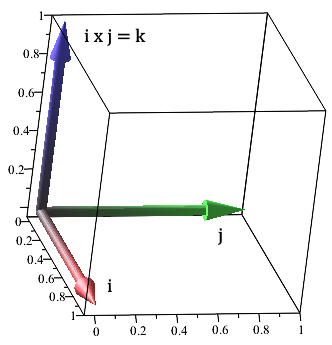See that $$\mathbf{i} \times \mathbf{j} = \left|\begin{array}{ccc} \mathbf{i} & \mathbf{j} & \mathbf{k} \\ 1 & 0 & 0 \\ 0 & 1 & 0 \end{array}\right| = \mathbf{k}$$

and by the anti-commutativity property of the cross product $$\mathbf{j} \times \mathbf{i} = -\left( \mathbf{i} \times \mathbf{j} \right) = -\mathbf{k}$$

The intersection of two planes

Since this topic is all about three-dimensional space, we look at planes as well as lines. A plane in three-dimensional $(x,y,z)$ space is a flat surface that can be expressed as $$f(x,y,z) = c$$

where $c$ is a constant value and the function on the left is linear with real coefficients. Picture it as an infinitely wide and long flat sheet. In FP3 you need to be able to work out if two planes intersect and if so, where. There are a few cases that can occur.

Line intersection is where the two planes intersect along an infinitely long line like this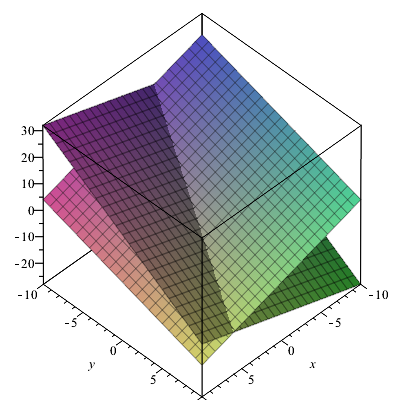They're parallel if they never meet once. They just carry on across the whole 3D space equidistant to each other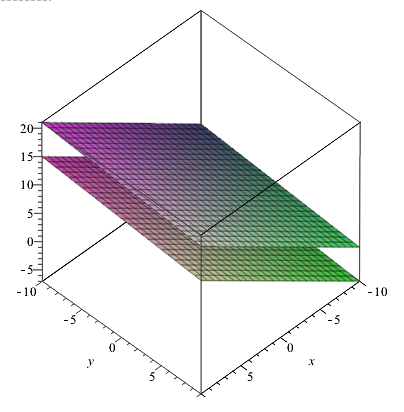They're coincident if they are the same plane. They meet at infinitely many points across the whole planeWe can describe these situations mathematically. Suppose you have two planes expressed by the following two equations with constant coefficients $a_i$ and $b_i$ \begin{align} a_1 x+a_2 y + a_3 z &= c \\ b_1 x+b_2 y + b_3 z &= d \end{align}

Then the normal vector to each plane is a vector that goes 90 degrees though the plane, consisting of the coefficients of $x$, $y$, and $z$ \begin{align} \mathbf{n}_1 &= a_1\mathbf{i}+a_2\mathbf{j} + a_3\mathbf{k} \\ \mathbf{n}_2 &= b_1\mathbf{i}+b_2\mathbf{j} + b_3\mathbf{k} \end{align}

Strictly speaking the normal vector is equal to what we call $\nabla f$, a vector consisting of the partial derivatives of $f(x,y,z)$. So for some plane expressed as $f(x,y,z) = c$ its normal vector is $$\mathbf{n} = \nabla f = \left(\frac{\partial f}{\partial x},\frac{\partial f}{\partial y},\frac{\partial f}{\partial z} \right)$$

There is a line intersection if the coefficients in the normal vectors satisfy $$\frac{a_1}{b_1} \ne \frac{a_2}{b_2} \ne \frac{a_3}{b_3}$$

The planes are parallel and never meet if the coefficients satisfy $$\frac{a_1}{b_1} = \frac{a_2}{b_2} = \frac{a_3}{b_3} \ne \frac{c}{d}$$

However they are coincident if they satisfy $$\frac{a_1}{b_1} = \frac{a_2}{b_2} = \frac{a_3}{b_3} = \frac{c}{d}$$

Here is the plane expressed by $x+y+z=1$ and its normal vector $(1,1,1)$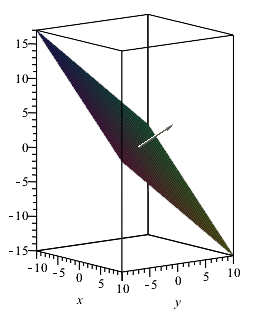If the normal vectors of two planes aren't parallel, then the two planes must meet. The cross product of these two normal vectors gives a vector which is perpendicular to both of them. Therefore the cross product is parallel to the line of intersection of the two planes. So this cross product will give a direction vector for the line of intersection.

If the normal vectors of two planes are parallel however, then by the properties of the cross product (and originally of a matrix determinant) if the two vectors are linearly dependent then the cross product will be zero. If the constant $c$ is the same for both planes, they are coincident so there are infinitely many intersection points. If not, they are parallel and they never meet.

So if the two planes aren't parallel and intersect along a line and the intersection direction vector has been found, we need to find the line equation for where they intersect. This is a position vector in the following form with the parameter $\lambda$ $$\mathbf{r}(\lambda) = \left(\textrm{starting position} \right) + \left(\textrm{intersect direction vector} \right)\lambda$$

The starting position can be any point shared by both the planes. This can be found by using simultaneous equations and picking a point.

Example

Q) Do the planes $x-2y+z = 1$ and $4x+y+z=4$ intersect? If so, state the line of points where they intersect.

A) The normal vectors of the two planes are $(1,-2,1)$ and $(4,1,1)$ respectively. The two lines are not parallel so the two planes meet. Find the direction of the intersection line by finding the cross product of the two normal vectors.

$$(1,-2,1) \times (4,1,1) = \left|\begin{array}{ccc} \mathbf{i} & \mathbf{j} & \mathbf{k} \\ 1 & -2 & 1 \\ 4 & 1 & 1 \end{array}\right| = \mathbf{i}\left(-2-1\right) - \mathbf{j}\left(1-4\right) + \mathbf{k}\left(1+8\right) = -3\mathbf{i} + 3\mathbf{j} + 9\mathbf{k}$$

Now we need to find a point where the two planes intersect. The two equations are \begin{align} x-2y+z &= 1 \\ 4x+y+z &=4 \end{align}

Subtracting the first equation from the second $$3x+3y=3 \Rightarrow x+y=1$$

Pick $x=1$ which means $y=0$. Then sub these values into the first equation

$$1 - 0 + z = 1 \Rightarrow z = 0$$

So a point is $(1,0,0)$. Therefore the line of intersection with the parameter $\lambda$ is $$\mathbf{r}(\lambda) = \left(1,0,0\right) + \left(-3,3,9\right)\lambda = \left(1-3\lambda,3\lambda,9\lambda\right)$$

The intersection of lines in three dimensions

As well as finding plane intersections, you need to be able to find the intersections of lines in three-dimensional space.

Recall from the last section that lines are expressed as $$\mathbf{r}(\lambda) = \left(\textrm{starting position} \right) + \left(\textrm{direction vector} \right)\lambda$$

From the work above you'll be able to see that if two lines with directions $\mathbf{a}$ and $\mathbf{b}$ are parallel they never intersect and $$\mathbf{a} \times \mathbf{b} = \mathbf{0}$$

However sometimes lines aren't parallel but they also don't intersect. This is easy to picture if you think of two thin straight lines in space. Such lines are skew. Their directions are linearly independent and therefore their cross product is non-zero.

If two vectors aren't parallel and do meet at some point then it is a simple matter of setting the lines equal to each other and solving for the parameter $\lambda$. We say that such lines are intersect.

Example

Q) Do the lines $\mathbf{r}_1 (\lambda) = (1+2\lambda,4\lambda,5-6\lambda)$ and $\mathbf{r}_2 (\lambda) = (\lambda,1+2\lambda,-3\lambda)$ intersect?

A) No because their direction vectors $(2,4,-6)$ and $(1,2,-3)$ are linearly dependent (one is twice the other), therefore they are parallel.

Example

Q) Do the lines $\mathbf{r}_1 (\lambda) = (2+5\lambda,1-4\lambda,4+\lambda)$ and $\mathbf{r}_2 (\lambda) = (1+\lambda,1-\lambda,-2\lambda)$ intersect?

A) No because although their direction vectors $(5,-4,1)$ and $(1,-1,-2)$ are linearly independent so they're not parallel, the three equations are inconsistent (they don't have a shared solution) \begin{align}2+5\lambda &= 1+\lambda \\ 1-4\lambda &= 1-\lambda \\ 4+\lambda &= -2\lambda \end{align} Therefore they are skew.

Example

Q) Do the lines $\mathbf{r}_1 (\lambda) = (1+\lambda,1-2\lambda,-2+9\lambda)$ and $\mathbf{r}_2 (\lambda) = (2-3\lambda,2\lambda,1-3\lambda)$ intersect?

A) Yes because their direction vectors $(1,-2,9)$ and $(-3,2,-3)$ are linearly independent so they're not parallel and there is a consistent solution to the three equations \begin{align} 1+\lambda &= 2-3\lambda \\ 1-2\lambda &= 2\lambda \\ -2+9\lambda &= 1-3\lambda \end{align} The solution is $\lambda = \frac{1}{4}$. Therefore the intersection point is $(\frac{5}{4},\frac{1}{2},\frac{1}{4})$.

Distance of a point from a line or from a plane

Another application of the cross product is in finding the shortest distance from a point to either a line or a plane.

Distance of a point from a line

The distance from a point $B$ to a line $\mathbf{r}$ is the smallest distance from the point to one of the infinitely many points on the line.

The vector from the original point $A$ of the line to the point $B$ is denoted $\overrightarrow{AB}$, and the direction vector of the line is called $\mathbf{d}$. The cross product of $\vec{AB}$ and $\mathbf{d}$ gives the normal vector with the correct direction. Then the distance we require is $$\frac{\|\overrightarrow{AB}\times\mathbf{d}\|}{\|\mathbf{d}\|}$$

This is the distance of the perpendicular line from the point to the line.

Example

Q) Calculate the shortest distance from the point $P(4,2,2)$ to the line $\mathbf{r}(\lambda) = (1+\lambda,\lambda,2\lambda-1)$.

A) From the line expression, point $A$ is at $(1,0,-1)$. The direction vector $\mathbf{d}$ is $(1,1,2)$. Therefore we can find $\overrightarrow{AP}$ $$\overrightarrow{AP} = (4,2,2)-(1,0,-1) = (3,2,3)$$

Then calculate the cross product of $\overrightarrow{AP}$ and $\mathbf{d}$ $$\overrightarrow{AP}\times\mathbf{d}=(3,2,3)\times(1,1,2) = \left|\begin{array}{ccc} \mathbf{i} & \mathbf{j} & \mathbf{k} \\ 3 & 2 & 3 \\ 1 & 1 & 2 \end{array}\right| = \mathbf{i}\left(4-3 \right) - \mathbf{j}\left(6-3\right) + \mathbf{k}\left(3-2\right) = \mathbf{i}-3\mathbf{j}+\mathbf{k}$$

The distance of the perpendicular line from the point to the line is therefore $$\frac{\|\overrightarrow{AP}\times\mathbf{d}\|}{\|\mathbf{d}\|} = \frac{\sqrt{1+\left(-3\right)^2+1}}{\sqrt{1+1+2^2}} = \frac{\sqrt{11}}{\sqrt{6}} = \frac{\sqrt{66}}{6}$$

Distance of a point from a plane

Given a point $B$ at $(x_0,y_0,z_0)$ and a plane $f(x,y,z)=c$ with equation $$a_1 x + a_2 y + a_3 z = c$$

and normal line $\mathbf{n}$, the shortest distance between the point and the plane is $$\frac{\left|f(x_0,y_0,z_0)-c\right|}{\|\mathbf{n}\|}=\frac{\left|a_1 x_0+a_2 y_0 +a_3 z_0-c\right|}{\sqrt{a_1^2+a_2^2+a_3^2}}$$

Example

Q) Calculate the shortest distance from the point $(-1,1,1)$ and the plane $x-5y+z=4$.

A) $$\frac{\left|-1-5 +1-4\right|}{\sqrt{1+\left(-5\right)^2+1}} = \frac{9}{\sqrt{27}} = \sqrt{3}$$

The scalar triple product

I'm going to show you what the scalar triple product means geometrically. Take the following calculation $$\left(2\mathbf{i}\times 2\mathbf{j}\right)\cdot 3\mathbf{k}$$

This is a scalar triple product that gives the volume of a cuboid with length 2, width 2, and height 3. First see that $$2\mathbf{i}\times 2\mathbf{j} = 4\mathbf{k}$$

This is the area of a rectangle of length 2 and width 2, but as a vector in the $\mathbf{k}$ direction. Then find the dot product of this and the height $3\mathbf{k}$ $$4\mathbf{k} \cdot 3\mathbf{k} = 12$$

giving the actual volume of the cuboid.

We can actually cycle through the three vectors and the scalar triple product gives the same results $$\left(2\mathbf{i}\times 3\mathbf{j}\right)\cdot 2\mathbf{k} = 6\mathbf{k}\cdot 2\mathbf{k}=12$$

If you think about it this is the same cuboid but with the length, width, and height switched around.

In general terms the scalar triple product of three vectors is a number, calculated as \begin{align} \left(\mathbf{a}\times \mathbf{b}\right)\cdot \mathbf{c} &= \left|\begin{array}{ccc} \mathbf{i} & \mathbf{j} & \mathbf{k} \\ a_1 & a_2 & a_3 \\ b_1 & b_2 & b_3 \end{array}\right|\cdot \mathbf{c} \\[1em] &= \left(\left|\begin{array}{cc} a_2 & a_3 \\ b_2 & b_3 \end{array}\right|,-\left|\begin{array}{cc} a_1 & a_3 \\ b_1 & b_3 \end{array}\right|,\left|\begin{array}{cc} a_1 & a_2 \\ b_1 & b_2 \end{array}\right|\right)\cdot\mathbf{c} \\[1em] &= \left|\begin{array}{ccc} c_1 & c_2 & c_3 \\ a_1 & a_2 & a_3 \\ b_1 & b_2 & b_3 \end{array}\right| \end{align}

The scalar triple product yields the same result when you cycle the vectors and operations \begin{align} \mathbf{a}\cdot\left(\mathbf{b}\times \mathbf{c}\right) &= \mathbf{a}\cdot\left|\begin{array}{ccc} \mathbf{i} & \mathbf{j} & \mathbf{k} \\ b_1 & b_2 & b_3 \\ c_1 & c_2 & c_3 \end{array}\right| \\[1em] &= \mathbf{a}\cdot\left(\left|\begin{array}{cc} b_2 & b_3 \\ c_2 & c_3 \end{array}\right|,-\left|\begin{array}{cc} b_1 & b_3 \\ c_1 & c_3 \end{array}\right|,\left|\begin{array}{cc} b_1 & b_2 \\ c_1 & c_2 \end{array}\right|\right) \\[1em] &= \left|\begin{array}{ccc} a_1 & a_2 & a_3 \\ b_1 & b_2 & b_3 \\ c_1 & c_2 & c_3 \end{array}\right| \end{align}

This works because when you switch two rows of a matrix, you multiply the determinant by -1. But we've switched two rows twice, keeping the determinant the same. Notice that we've kept the rows representing the elements of $\mathbf{a}$, $\mathbf{b}$, and $\mathbf{c}$ in the same order to cycle them around. Also notice by the symmetry of the dot product

$$\left(\mathbf{a}\times \mathbf{b}\right)\cdot \mathbf{c} = \mathbf{c}\cdot\left(\mathbf{a}\times \mathbf{b}\right)$$ $$\mathbf{a}\cdot\left(\mathbf{b}\times \mathbf{c}\right) = \left(\mathbf{b}\times \mathbf{c}\right)\cdot\mathbf{a}$$

Volume of a parallelepiped and a tetrahedron

Geometrically, the absolute value of the scalar triple product represents the volume of a parallelepiped whose main edges are three vectors $\mathbf{a}$, $\mathbf{b}$, and $\mathbf{c}$ that meet in the same vertex.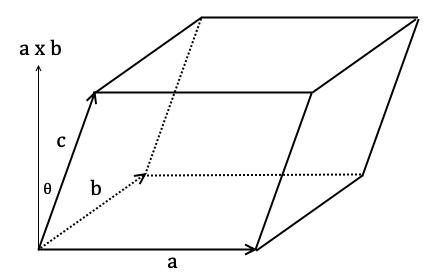Given the angle between $\mathbf{a}\times \mathbf{b}$ and $\mathbf{c}$ is $\theta$, the volume is $$\|\mathbf{a}\times \mathbf{b}\|\|\mathbf{c}\|\cos\theta = \left(\mathbf{a}\times \mathbf{b}\right)\cdot\mathbf{c} = \left|\begin{array}{ccc} c_1 & c_2 & c_3 \\ a_1 & a_2 & a_3 \\ b_1 & b_2 & b_3 \end{array}\right|$$

Example

Q) Find the volume of the parallelepiped with three main edges $(2,0,0)$, $(1,2,0)$, and $(1/2,1/2,2)$.

A) Let $\mathbf{a} = (2,0,0)$, $\mathbf{b} = (1,2,0)$, and $\mathbf{c} = (1/2,1/2,2)$. Then the scalar triple product is $$\left(\mathbf{a}\times \mathbf{b}\right)\cdot \mathbf{c} = \left|\begin{array}{ccc} \frac{1}{2} & \frac{1}{2}& 2 \\ 2 & 0 & 0 \\ 1 & 2 & 0 \end{array}\right| = 2\left(4-0\right) = 8 ~\textrm{units}^3$$

The volume of a tetrahedron is equal to a sixth of the absolute value of the scalar triple product. Recall that a tetrahedron is a regular triangular pyramid composed of four equally sized equilateral triangles.Given the angle between $\mathbf{a}\times \mathbf{b}$ and $\mathbf{c}$ is $\theta$, the volume is $$\frac{1}{6}\|\mathbf{a}\times \mathbf{b}\|\|\mathbf{c}\|\cos\theta = \frac{1}{6}\left(\mathbf{a}\times \mathbf{b}\right)\cdot\mathbf{c} = \frac{1}{6}\left|\begin{array}{ccc} c_1 & c_2 & c_3 \\ a_1 & a_2 & a_3 \\ b_1 & b_2 & b_3 \end{array}\right|$$

The shortest distance between two skew lines

If two straight lines are skew then there must be a point at which the distance between them is at a minimum. If two lines are defined as each having a starting point and a direction vector, then we'll call their starting points are $A$ and $B$ respectively, and their direction vectors $\mathbf{d}$ and $\mathbf{e}$ respectively.

Then the shortest distance between the two skew lines is the absolute value of the scalar triple product of the direction vector $\overrightarrow{BA}$ and the unit vector in the direction of the cross product of $\mathbf{d}$ and $\mathbf{e}$.

$$\left| \overrightarrow{BA}\cdot\frac{\left(\mathbf{d}\times\mathbf{e}\right)}{\left|\mathbf{d}\times\mathbf{e}\right|} \right|$$

Condition for two lines to intersect

The formula for the minimum distance between two lines is

$$\left| \overrightarrow{BA}\cdot\frac{\left(\mathbf{d}\times\mathbf{e}\right)}{\left|\mathbf{d}\times\mathbf{e}\right|} \right|$$

Therefore two lines must intersect if this minimum distance is equal to zero, i.e.

$$\left| \overrightarrow{BA}\cdot\frac{\left(\mathbf{d}\times\mathbf{e}\right)}{\left|\mathbf{d}\times\mathbf{e}\right|} \right| = 0$$

By the definition of the dot product, if $\theta$ is the angle between the lines

$$\|\overrightarrow{BA}\|\left\Vert\frac{\left(\mathbf{d}\times\mathbf{e}\right)}{\left|\mathbf{d}\times\mathbf{e}\right|}\right\Vert\cos\theta = 0$$

If the two non-parallel lines intersect in 3D space then they must be coplanar. If you imagine two thin straight lines in 3D space, then it makes sense that they both must lie on some hypothetical flat plane for them to intersect.

Therefore the two vectors $\overrightarrow{BA}$ and $\left(\mathbf{d}\times\mathbf{e}\right)$ must be at right angles to each other. By the definition of the dot product that I wrote above, their dot product is therefore equal to 0 since $\cos\frac{\pi}{2}=0$.

Additionally if the two lines have the same starting point then $\overrightarrow{BA} = \mathbf{0}$ which also zeroes the expression, since they intersect at the shared starting point.

However if two lines are parallel and do intersect then they must necessarily share the same starting point to intersect. In this case they are coincident lines and the cross product of their direction vectors is zero. There are infinitely many intersection points along the lines. Otherwise they never intersect but the cross product of their direction vectors is still zero.